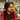# Number of Islands

Given an m x n 2D binary grid grid which represents a map of ‘1’s (land) and ‘0’s (water), return the number of islands.

An island is surrounded by water and is formed by connecting adjacent lands horizontally or vertically. You may assume all four edges of the grid are all surrounded by water.

## Example:

`````` Input: [
["1","1","1","1","0"],
["1","1","0","1","0"],
["1","1","0","0","0"],
["0","0","0","0","0"]
]
Output: 1``````
``````Input: [
["1","1","0","0","0"],
["1","1","0","0","0"],
["0","0","1","0","0"],
["0","0","0","1","1"]
]
Output: 3``````

## Solution:

``````var numIslands = function (grid) {
var m = grid.length
var n = grid.length
var count = 0
for (var i = 0; i < m; i++) {
for (var j = 0; j < n; j++) {
if (grid[i][j] == "1") {
count++
bfs(i, j)
}
}
}
function bfs(i, j) {
var que = [[i, j]]
while (que.length != 0) {
var el = que.shift()
if (grid[el][el] == "0") continue
grid[el][el] = "0"
var dirs = [
[1, 0],
[-1, 0],
[0, 1],
[0, -1],
]
for (var dir of dirs) {
var x = el + dir
var y = el + dir
if (x >= 0 && x < m && y >= 0 && y < n && grid[x][y] == "1") {
que.push([x, y])
}
}
}
}
return count
}``````Written by Gagandeep Rangi who likes to talk about himself in third person. Twitter Instagram